# Algebra - problems - page 45

1. Outer anglesThe outer angle of the triangle ABC at the A vertex is 71°40 ' outer angle at the vertx B is 136°50'. What size has the inner triangle angle at the vertex C?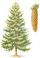On a weekly forest brigade operates 12 students. After plant one hundred spruces get x CZK, anfter one hundred pines y CZK. How many CZK got one student for one day if planted spruces 25000 and 30000 pines week?
3. Prism basesVolume perpendicular quadrilateral prism is 360 cm3. The edges of the base and height of the prism are in the ratio 5:4:2 Determine the area of the base and walls of the prism.
4. Translations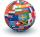If I going to translate the book 6 pages per day I translate it 4 days earlier than if I translated 5 pages a day. If I translate 4 pages a day I translate it for how many days.....?
5. Mason with assistantMason had to complete the work in 10 days. Two and a half days later his assistant arrived. Together they completed the work in next three and a half days. How many days would he need an assistant for the same work?
6. Float boya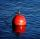A 0.5 meter spherical float is used as a location mark for a fishing boat anchor. It floats in salt water. Find the depth to which the float sinks if the material of which the float is made weighs 8 kilograms per cubic meter and salt water weighs 1027 kg/m
7. Work at plant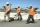Handyman has vowed to do repair work at the plant for 25 days. However, work had to be shortened and therefore gained helper. Together they made all the corrections for 13 1/3 days. How long the work would take helper himself?
8. Four classses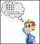Students of all 7, 8 and 9 classes in one school may take up 4,5,6 and 7 abreast and nobody will left. How many is the average count of pupils in one class if there are always four classes each grade?
9. BonbonsCreate a mixture of 50 kg of candy on price 700Kč. Candies has prices: 820Kč, 660Kč and 580Kč. Use cross rule.
10. Sisters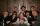Sisters Janka and Danka are saved together 220 CZK. On a trip to Janka wants to take a fifth of her savings and Danka quarter. Then they will have 50 CZK on the trip. How much money saved Janka and Danka?
11. Rabbit family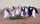Rabbit family ate 32 pieces of carrots, small one ate 6 pieces, dad 5 more than the mother. How much ate mom?
12. Percentage reduction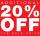Reducing the unknown number by 19% get number 700.7 Determine unknown number.
13. TrapeziumThe length of the base and the height size of the base of the trapezium is at ratio 5:3:2, the content area of the trapezium is 128 cm2. Calculate the length of the base and the height of the trapezoid.
14. EquationSolve equation and check the result: 1.4x - 3/2 + x - 9,8 = x + 0,4/3 - 7 + 1,6/6
15. Triangle ABCIn a triangle ABC side b measure 10 cm less than the side a and side b is half of the side c. Calculate the length of sides if the circumference of the triangle is 42 cm.
16. Resort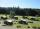In a completely crowded resort was 30% from the Czech Republic quarter visitors were from Slovakia and the rest 135 came from Germany. What capacity have resort?
17. Triangle midpoints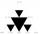Determine coordinates of triangle ABC vertices if we know tirangle sides midpoints SAB [0;3] SBC [1;6] SAC [4;5], its sides AB, BC, AC.
18. Three co-owners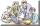The three co-owners of building company have earnings from a contract portioned in the ratio of 3:6:7. Each of them received the amount in whole USD. One of them on contract earned 86450 USD. What was the total earnings this order?
19. PrismCalculate the height of the prism having a surface area 448.88 dm² wherein the base is square with a side of 6.2 dm. What will be its volume in hectoliters?
20. Ski class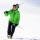Class attend 30 boys and some number of girls. Ski training was attended by 28 boys and all the girls, which was 95% of all students. How many girls attend this class? How many percent is that?

Do you have an interesting mathematical problem that you can't solve it? Enter it, and we can try to solve it.

To this e-mail address, we will reply solution; solved examples are also published here. Please enter e-mail correctly and check whether you don't have a full mailbox.